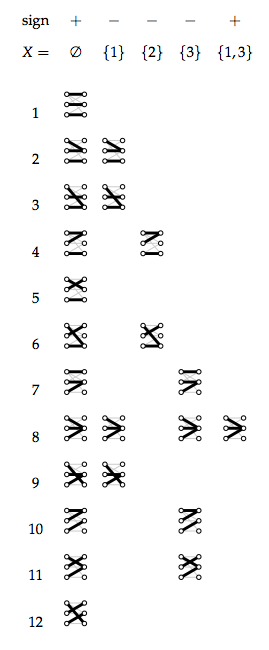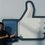# When Math meets CS: Counting Perfect Matchings using Inclusion ExclusionI'm sure you've all heard of buzz-words like NP-Completeness and the like. They sound hard and unapproachable, so today let's solve one of the non-classical NP-Hard problems (in exponential time of course).

A graph, $G$, is basically a set of points (called vertices) on a plane floating around. However, there are also a set of lines joining the various points together in order to relate them together somehow. These are called nodes and edges, to imagine them, you can think of the cliche social network graph with the dense set of edges connecting you to possibly thousands of friends and acquantences. Typically, graphs aren't really that dense and well-connected, and typically you'll only see very few edges floating around. More formally, we describe a graph $G$ by a pair of sets: $V$, standinf for vertices; $E$ standing for edges. Each edge is composed of a line and two vertices, and we may denote the edge going from $u$ to $v$ (both vertices) as $u - v$. (Note in this presentation, edges do not have a direction.)Altbipartite graph

Now, the problem of constructing a perfect matching turns out to be relatively easy to do with pen and paper, and even more so by a computer (we won't go into that right now, wait until your first algorithm course). However, not satisfied with just one perfect matching, I want you to tell me how many perfect matchings there are in a bipartite graph! As far as we know, this is a really hard problem.

How would you solve this problem? A natural starting point would be to brute-force the solution; since the problem is hard, we can't expect a sub-exponential algorithm anyways! Fair point, let's start with a brute-force algorithm. Suppose your bipartite graph has $m = |E|$ edges and $n = |V|$ nodes (where $n$ is even by definition of $G$ being bipartite), then a perfect matching must be a set of $\frac{n}{2}$ edges. One way to solve this problem then is to enumerate all of the ${m \choose n/2}$ such edges, test each one individually to see if it is a perfect matching, and count how many were actually perfect matchings. How long would this algorithm take? Well, suppose $m = c\frac{n}{2}$ for $c \ge 1$ (because otherwise we can't have a perfect matching) and since there are ${m \choose n/2}$ such edges we have to consider, then it's possible to show that the number of edge sets we have to consider is approximately $\sqrt{c}^n$! For many applications, it's very likely that $c$ isn't small. For example, consider the realestate market, here, it's not at all unlikely that the average number of houses considered per potential buyer is greater than 4! Can we do better?

Since in this problem, the number of edges could be potentially up to $O(m^2)$, it's a very desirable property to construct an algorithm that is completely independent of $m$ the number of edges! It turns out that there's a really elegant algorithm (that can be further improved in fact) which runs in time $O(2^n)$, no matter how many edges there are in our graph. Here's how to do it.

Consider the inclusion-exclusion principle, and let's consider a generalization of it. Suppose we have a function $f(X \subseteq V): \text{subssets}(V) \to \mathbb{N}$ that assigns a natural value to each subset of $V$, then we let the zeta-transform of $f(X)$ to be $\zeta f = g(V) = \sum_{X \subseteq V} f(X)$. A generalization of the Mobius Inversion Theorem (lifted out of number theory and applied to complete partial orders) states that $g(V) = \sum_{X \subseteq V} f(X) \implies f(V) = \sum_{X \in V} (-1)^{|V - X|} g(X)$ which can be seen as a generalization of the inclusion/exclusion principle on arbitrary predicate function $f(X)$. Informally, you can easily see the relationship from the mobius inversion theorem to the inclusion-exclusion theorem, which states that $|V| = \sum_{X \in V} (-1)^{|V - X|} |X|$

Now, let's take a leap of faith together. Let $R$ be the right half of the vertices; define a semi-leftmatch to be a set of $n/2$ edges, each of which starts at exactly one vertex on the left side (so it is a perfect matching for only the left hand side). For example, this is a semi-leftmatch

<center>Alt text </center>

Now, define $g(X \subseteq R)$ to be the number of semi-leftmatches whose edges do not enter the right hand side vertices in $X \subseteq R$. For example, the above semi-leftmatch is also included in $g(\{a\})$. Then it turns out that $g(X)$ is the zeta transform of $f(X)$ that returns the number of perfect matchings when $X = R$, that is $g(R) = \zeta f(X) = \sum_{X \subseteq R} f(X) \implies f(R) = \sum_{X \subseteq R} (-1)^{(n/2 - |X|)} g(X)$ To see this is true, look at the following example:

<center>Alt text </center>

Here, we have $|R|$ = 3. Now, $g({})$ considers every possible semi-leftmatch, of which there are $3 \times 2 \times 2$ possibilities (the first left node has 3 edges, the second has 2, and the last also has 2). Of course, we also need to subtract off the non-matching semimatches. Notice that we have the invariant: if we exclude a node out of the right hand side for matching, then none of the semimatches generated are true matchings, because it's impossible to match $n$ left hand side nodes with $n-1$ right hand side nodes. Now, if you actually look closely, there are only 3 perfect matchings. If you do the mobius inversion on $g$, which you can compute by counting the number of semimatches (shown in the figure), you'll also get 12 - 4 - 2 - 4 + 1 + 0 + 0 + 0 = 3! (I neglected to include the cases where there are zero semimatches).

Therefore, in order to compute the number of perfect matchings, we just need $2^{|R|}$ computations of $g(X)$, which is easy:

function g(G,X)
remove from E all edges pointing to each node of X
remove from R all of X
return the product of the number of remaining edges coming out of each of the node on the left
end


that is, collapse $G$ into $\hat G = (V, \hat E) = G \backslash X$ by removing all of the nodes and edges connected to $X$, then $g(X) = \prod_{l \in L} \text{deg}_{\hat E}(l)$ where $\text{deg}(v)$ is the function that returns the number of edges connected to a vertex $v$. We can then use the mobius inversion transform above to compute $f(R)$ in $O(\sqrt{2}^n)$ time, irregardless of how many edges are present.

This concludes another presentation on using clever mathematics to solve problems in computer science. The algorithm derived here came from relatively recent research, and as of late, inclusion-exclusion has been an actively researched area in algorithm design. For those interested, I would recommend Fast polynomial-space algorithms using M ̈obius inversion: Improving on Steiner Tree and related problemsNote by Lee Gao
6 years, 10 months ago

This discussion board is a place to discuss our Daily Challenges and the math and science related to those challenges. Explanations are more than just a solution — they should explain the steps and thinking strategies that you used to obtain the solution. Comments should further the discussion of math and science.

When posting on Brilliant:

• Use the emojis to react to an explanation, whether you're congratulating a job well done , or just really confused .
• Ask specific questions about the challenge or the steps in somebody's explanation. Well-posed questions can add a lot to the discussion, but posting "I don't understand!" doesn't help anyone.
• Try to contribute something new to the discussion, whether it is an extension, generalization or other idea related to the challenge.

MarkdownAppears as
*italics* or _italics_ italics
**bold** or __bold__ bold
- bulleted- list
• bulleted
• list
1. numbered2. list
1. numbered
2. list
Note: you must add a full line of space before and after lists for them to show up correctly
paragraph 1paragraph 2

paragraph 1

paragraph 2

[example link](https://brilliant.org)example link
> This is a quote
This is a quote
    # I indented these lines
# 4 spaces, and now they show
# up as a code block.

print "hello world"
# I indented these lines
# 4 spaces, and now they show
# up as a code block.

print "hello world"
MathAppears as
Remember to wrap math in $$ ... $$ or $ ... $ to ensure proper formatting.
2 \times 3 $2 \times 3$
2^{34} $2^{34}$
a_{i-1} $a_{i-1}$
\frac{2}{3} $\frac{2}{3}$
\sqrt{2} $\sqrt{2}$
\sum_{i=1}^3 $\sum_{i=1}^3$
\sin \theta $\sin \theta$
\boxed{123} $\boxed{123}$

Sort by:

Typo, second paragraph: "$V$, standinf for..." should be "standing for".

- 6 years, 10 months ago

I think I am somewhat confused. I couldn't read till the end because I lost in between. So in the bipartite graph you are allowing edges inside the same group also.. isn't it? Why cant we have perfect matching with c=1? Why isn't the total number of perfect matchings be equal to n! ?

- 6 years, 10 months ago

1. No a bipartite graph only allows edges from the left group into the right group. It has a characteristic look of two disjoint set of vertices.

2. Oops, I meant $m = \frac{cn}{2}$ and considering $c \ge 1$. The case of $c = 1$ is the trivial ladder shaped graph.

3. Recall that a matching matches one node in the left group into a unique node into the right. However, recall also that matchings can only be made on edges that are already included as part of our bipartite graph. We're not allowed to add in arbitrary edges. In order to construct $n!$ perfect matchings in a bipartite graph with $2n$ nodes ($n$ in the left and $n$ in the right), it better be the case that each perfect matching represents a mapping from the left group into some permutation on the right, which means that it must be possible to match ever node in the left with every node in the right: this is what we will call a complete bipartite graph. However, not all bipartite graphs are complete, the example I used up there cannot make $n!$ maximum matchings.

- 6 years, 10 months ago

Thanks for the quick reply! Can you then fix that $m=cn$ in the article? Also now I think I understand this problem and I will read it neatly. :-)

Actually I work in related field (theory of complex networks) and I am actually writing a series about it on brilliant (https://brilliant.org/newsfeed/tag-feed/complexnetworks/). If you want, we can try to make our articles complementary!

- 6 years, 10 months ago

Thanks, I've made the changes :) if you find any other mistakes please let me know as well. I didn't get much of a chance to proof-read this article before posting it under time constraint last night.

I'm also interested in network theory, albeit because my background has been very combinatorial in nature, I enjoy analyzing game theoretic and combinatorial properties of network behavior: matching mechanisms, incentive properties, and algorithm design for various graph problems, but I haven't had much of a chance to look at the more analytic side on properties of networks themselves and have been attempting to look into these, which seems to be what your articles focus on. This seems like a great pairing then :) I would love to publish complementary articles on the subject :)

- 6 years, 10 months ago

if you like, we can communicate over email: snehalshekatkar@gmail.com

- 6 years, 10 months ago

Okay :) mine is lg342@cornell.edu

- 6 years, 10 months ago